# Madhya Pradesh Board Question Paper for Class 10th Maths 2018 In PDF

## MP Board (MPBSE) Question Paper 2018 Class 10th Mathematics For English Medium with Solutions – Free Download

### MPBSE Class 10th Maths Question Paper With Solutions 2018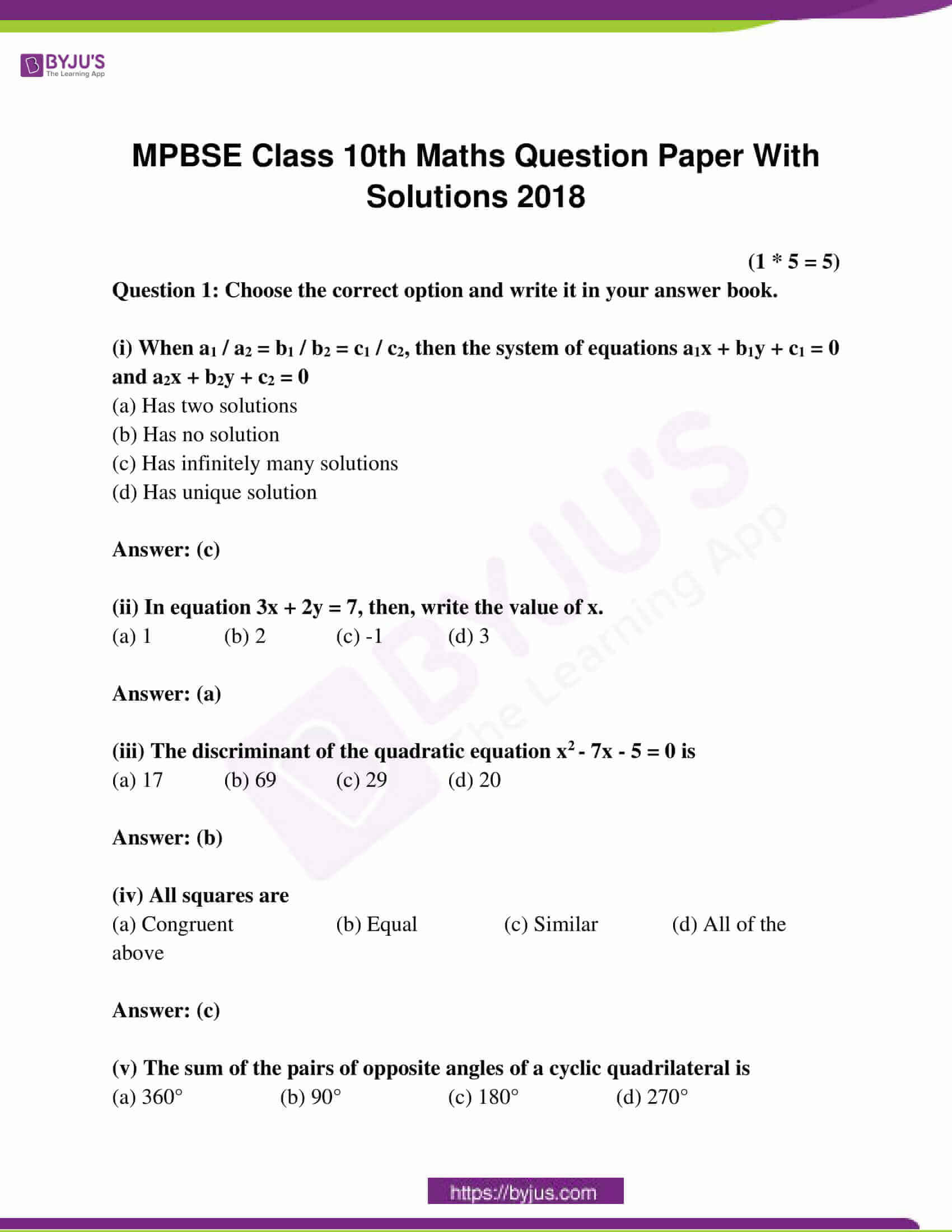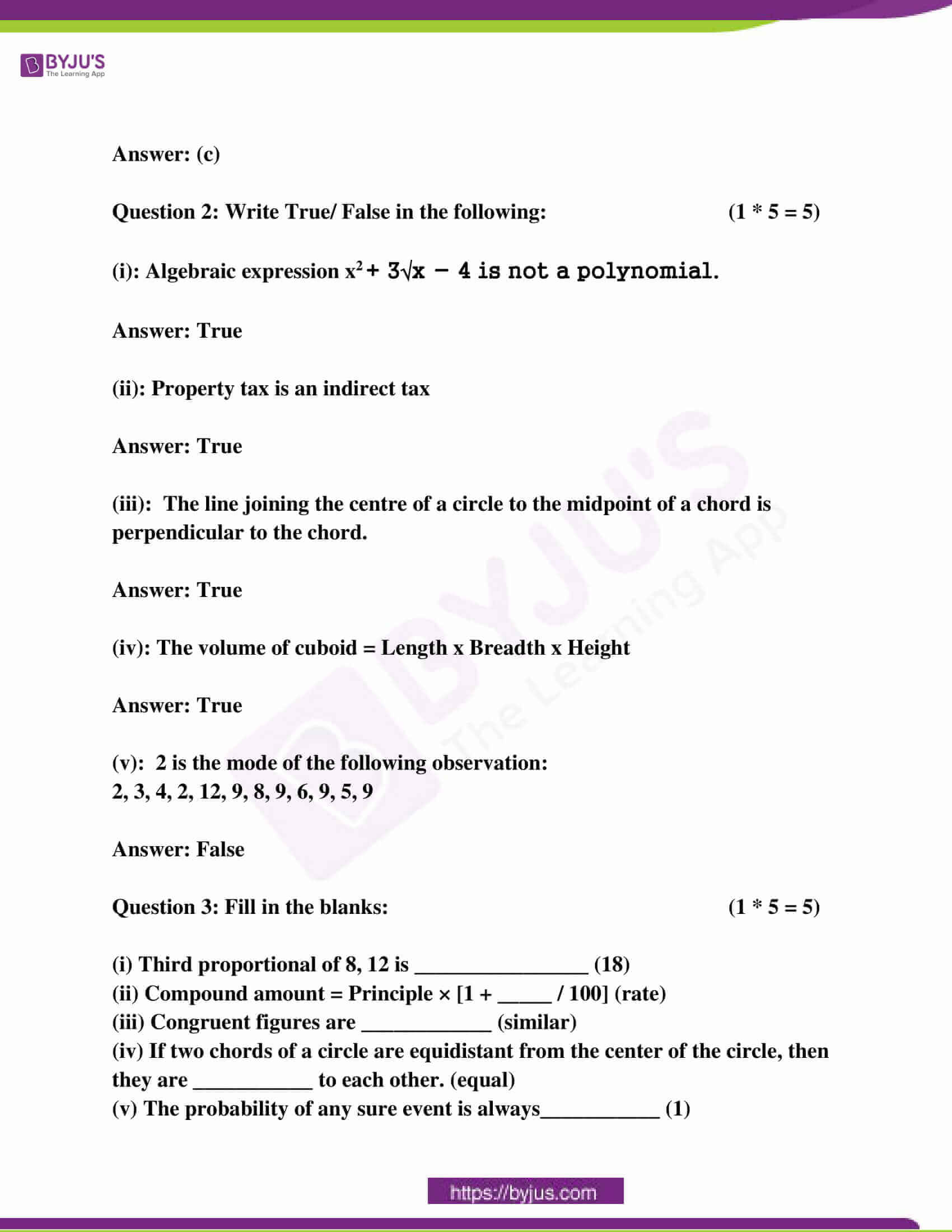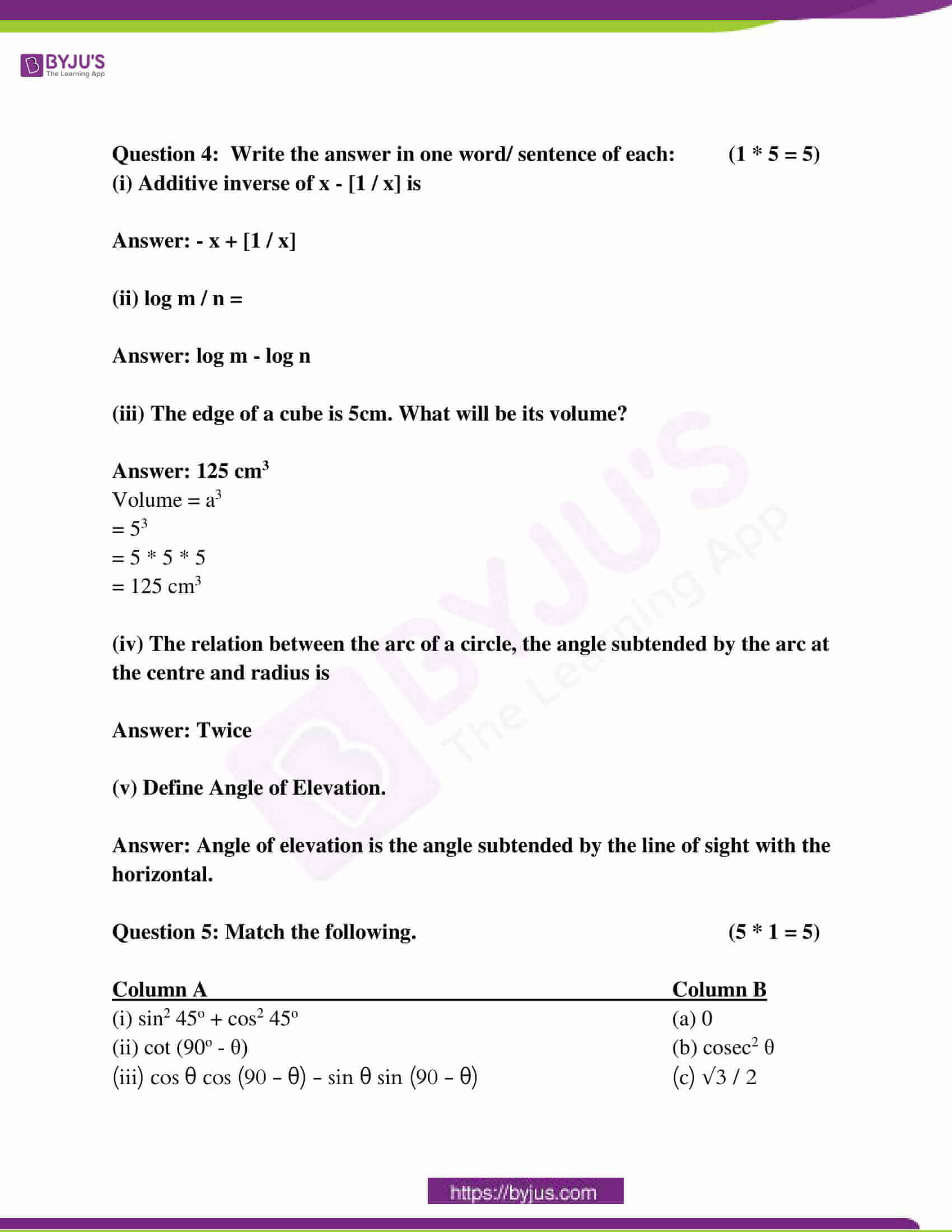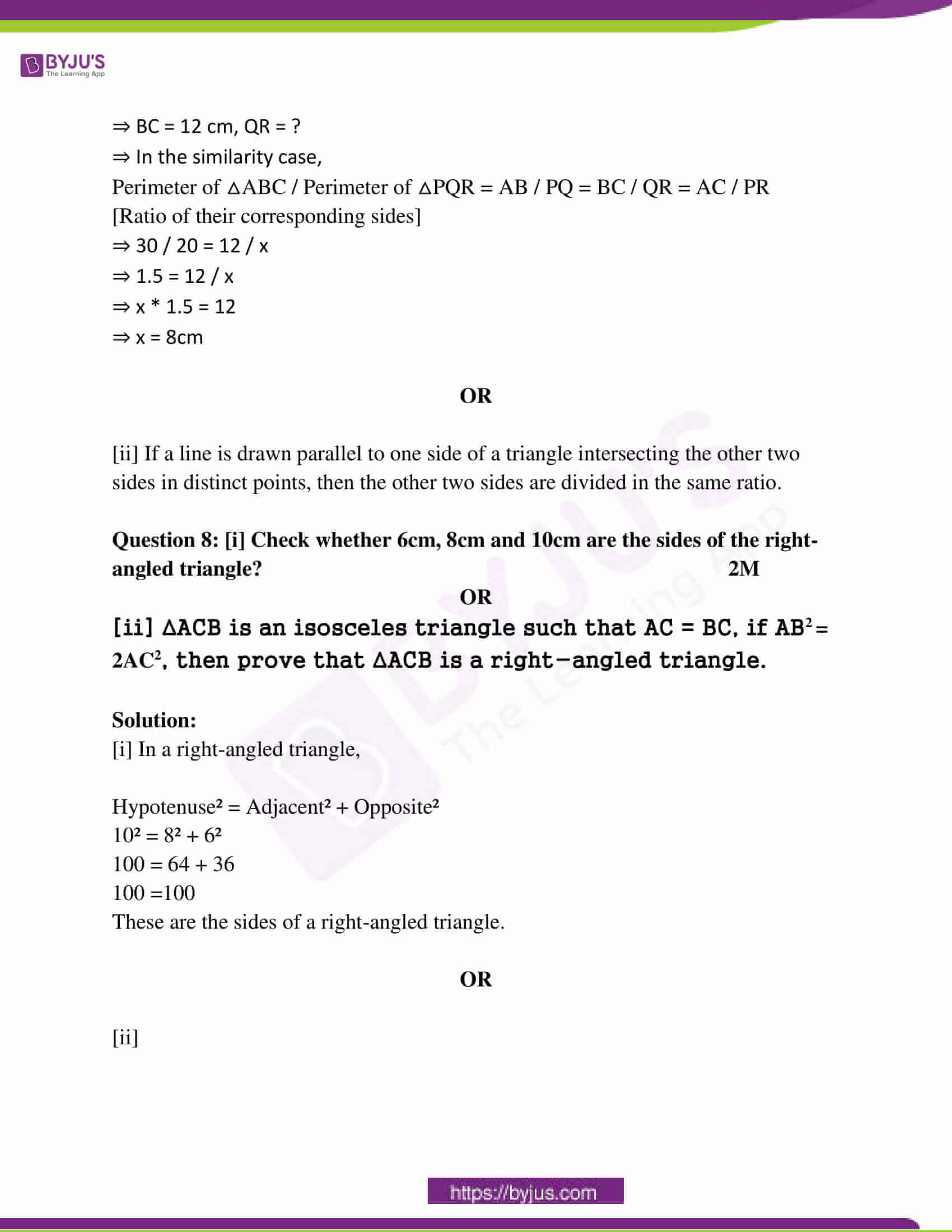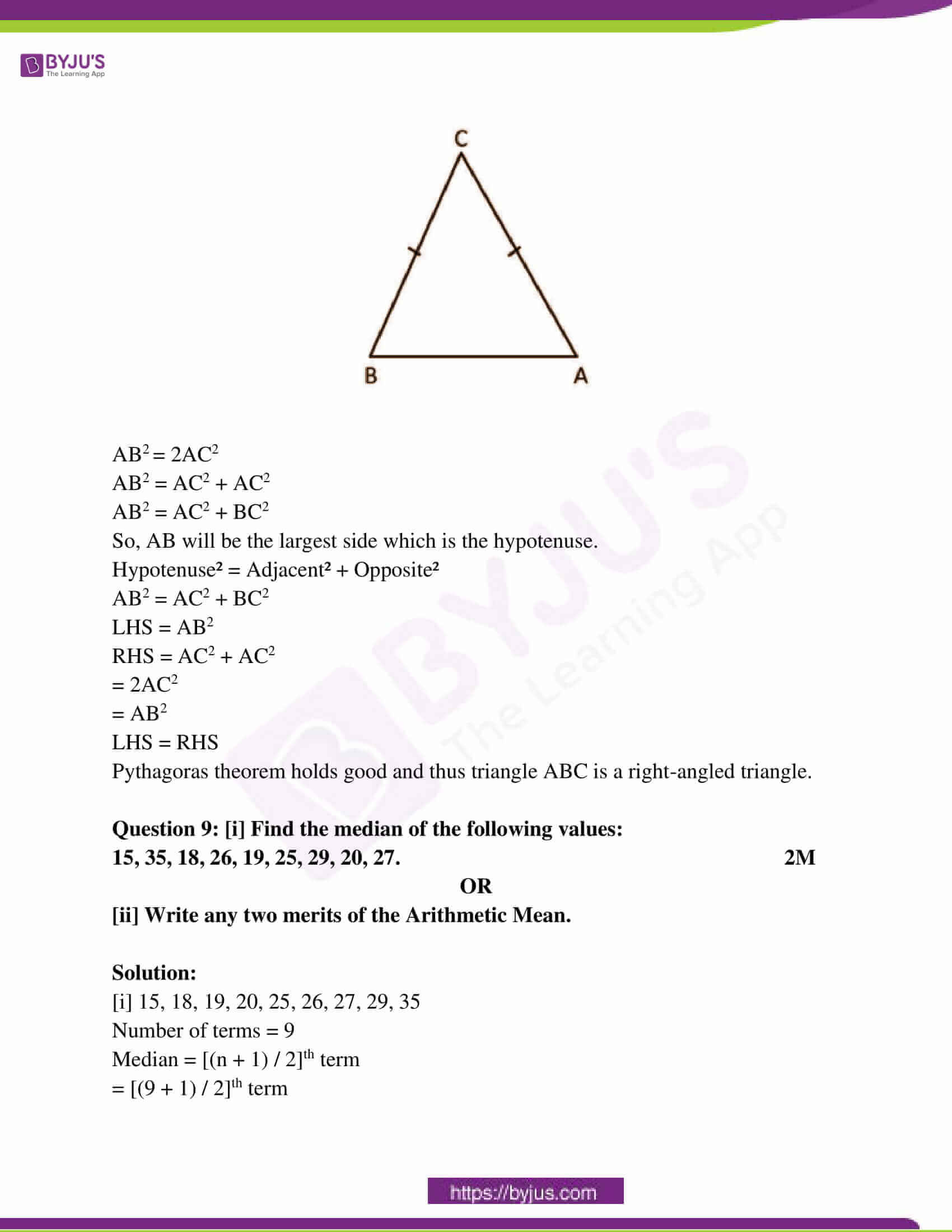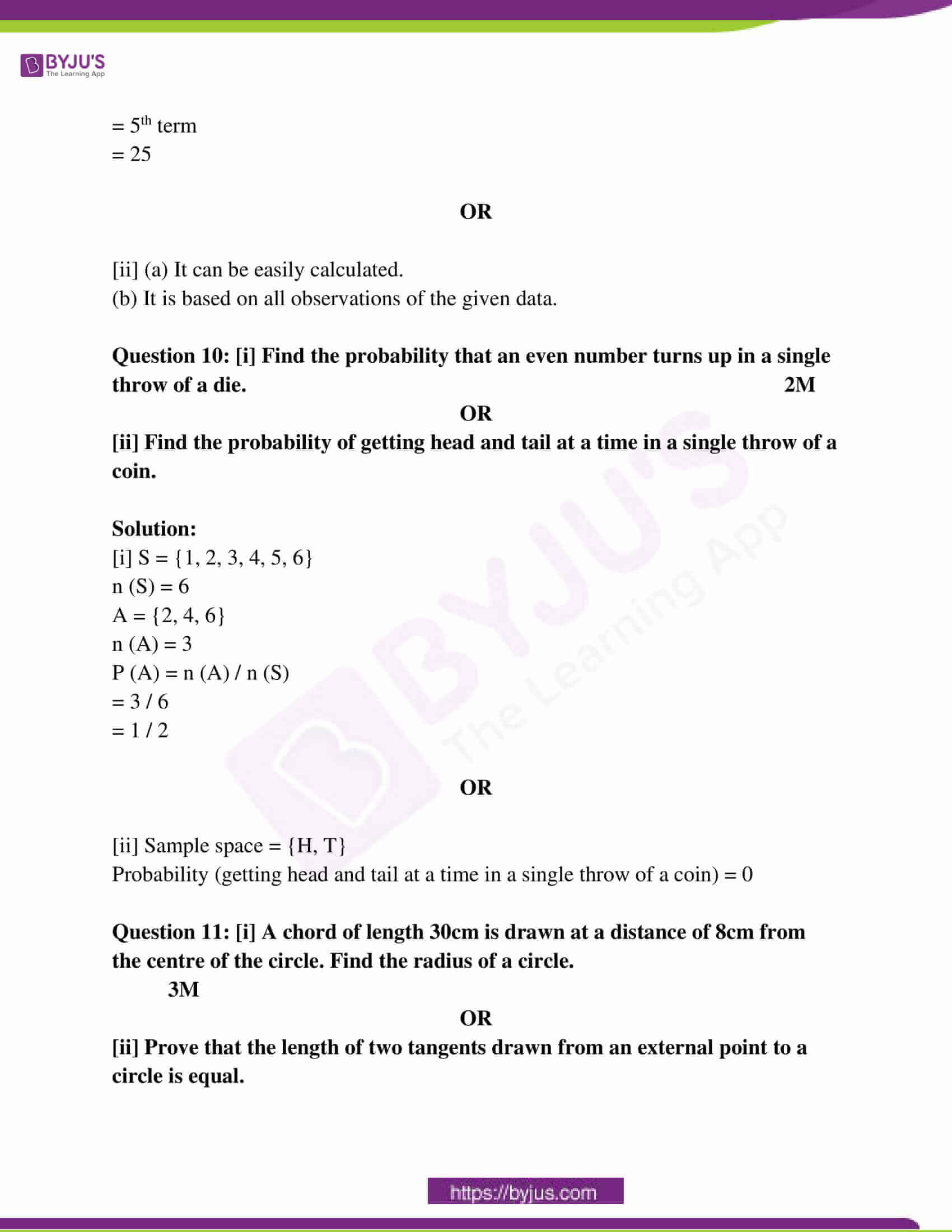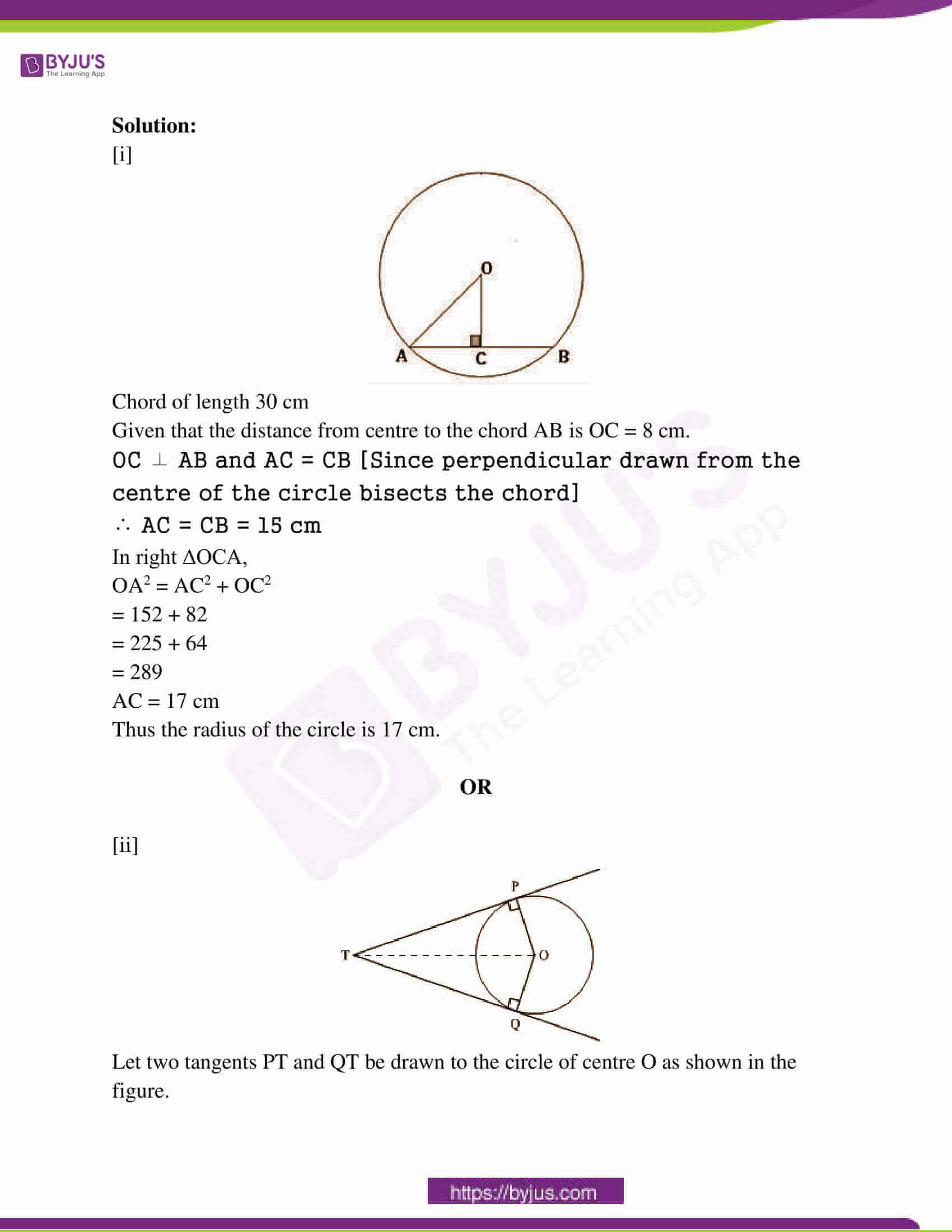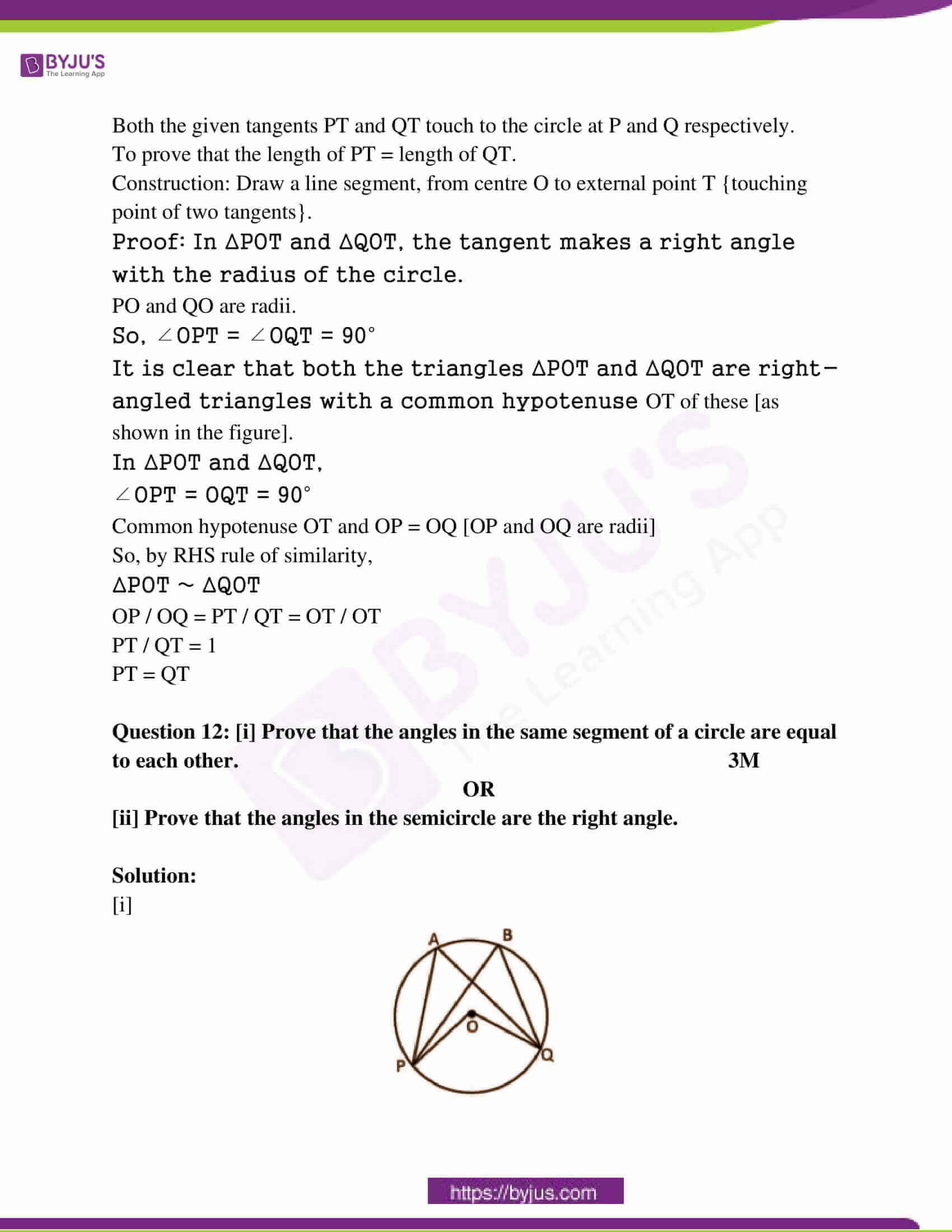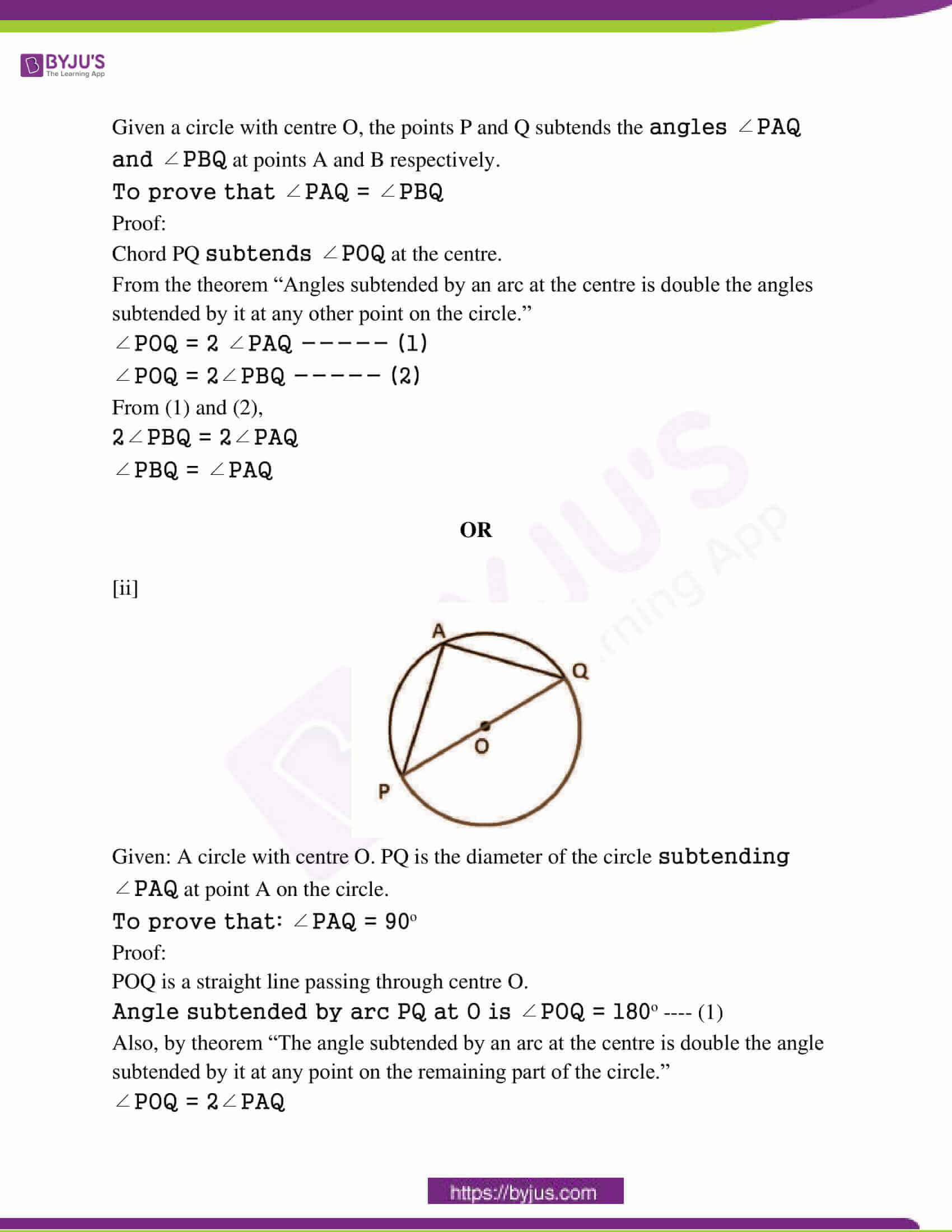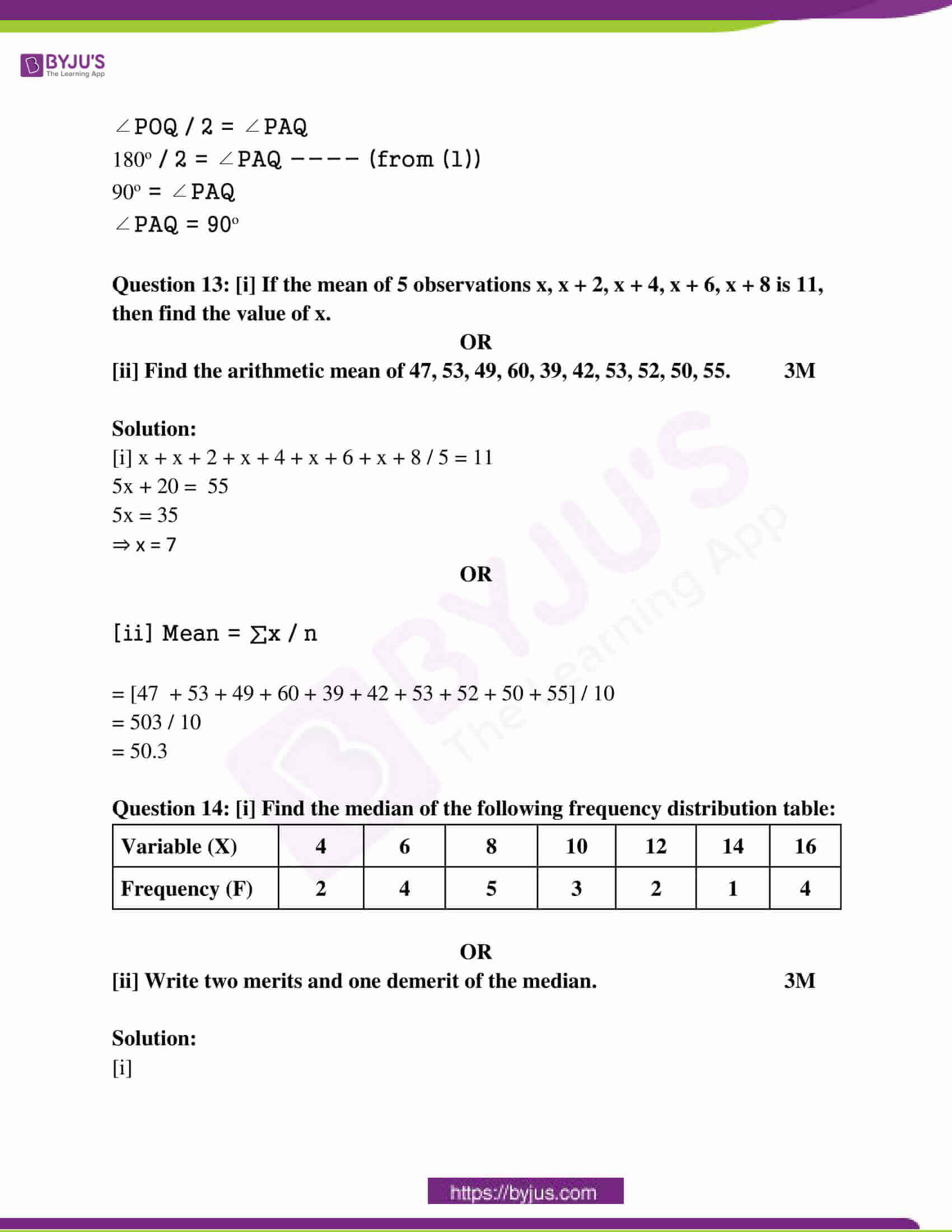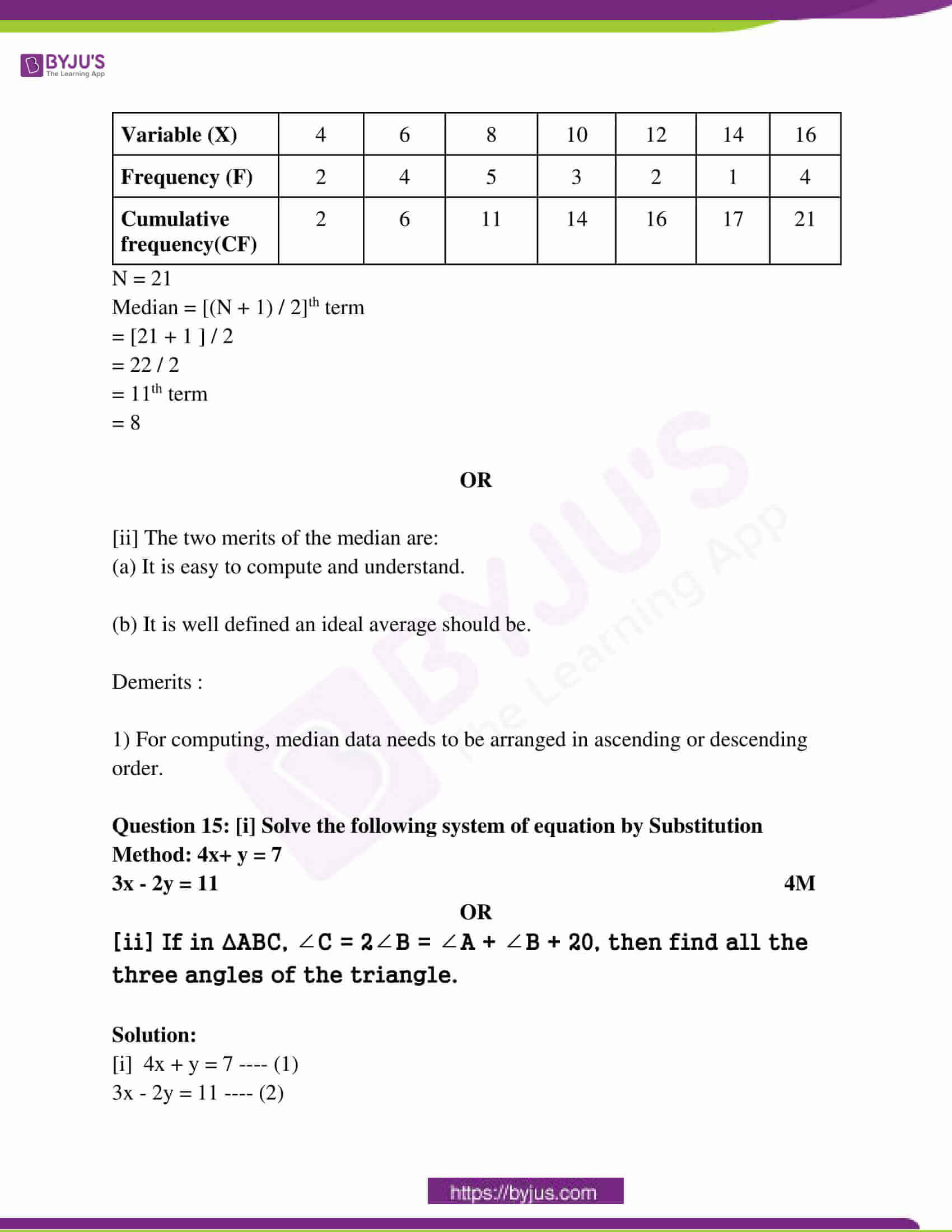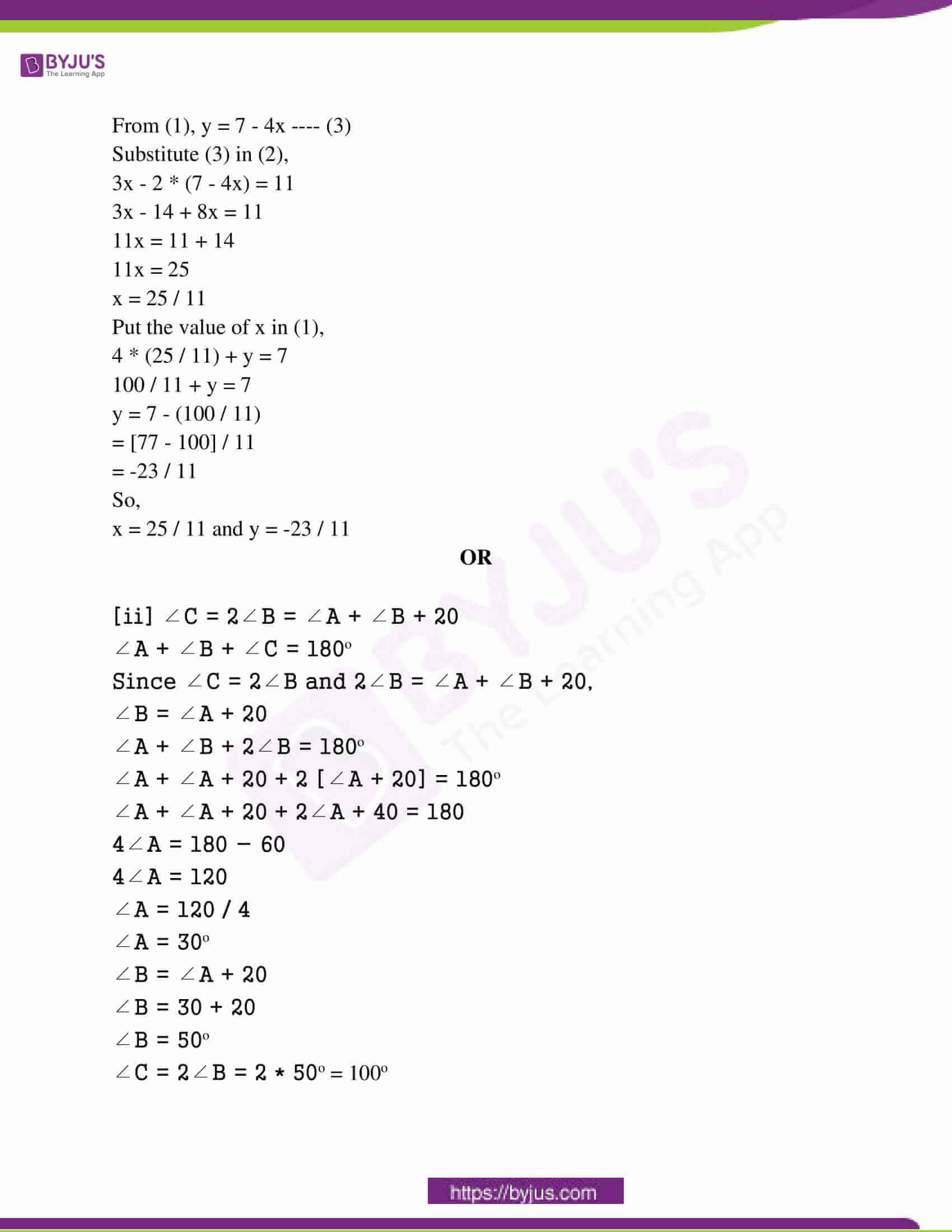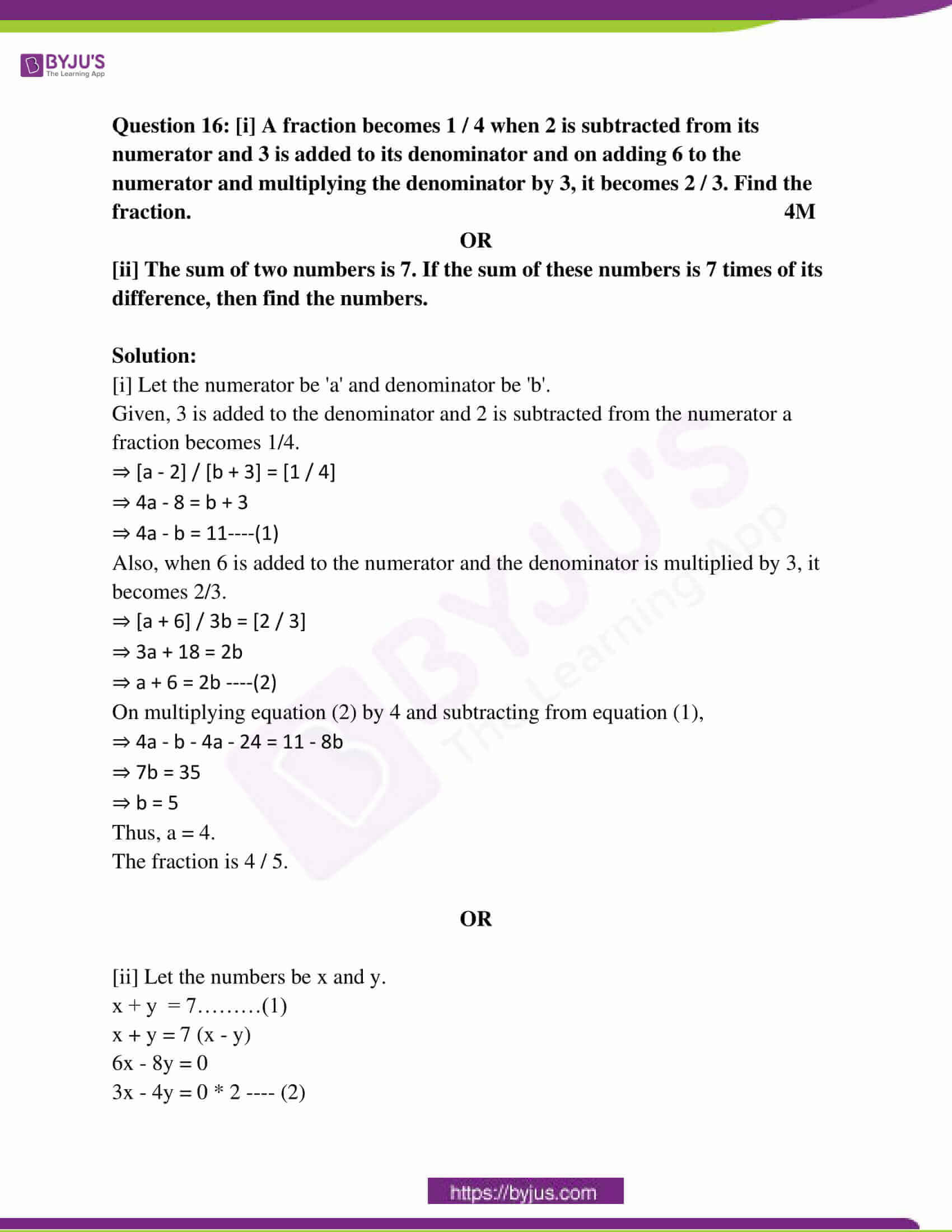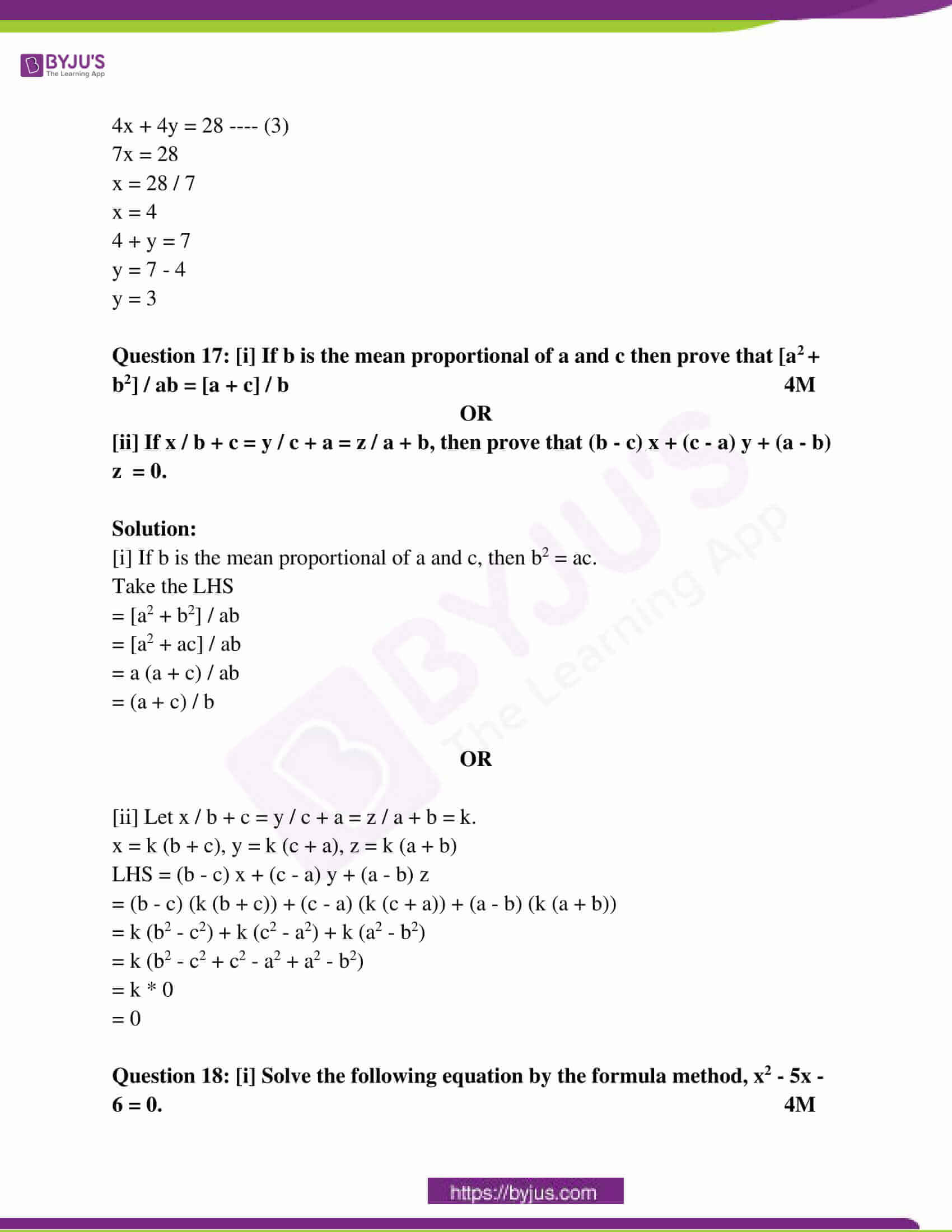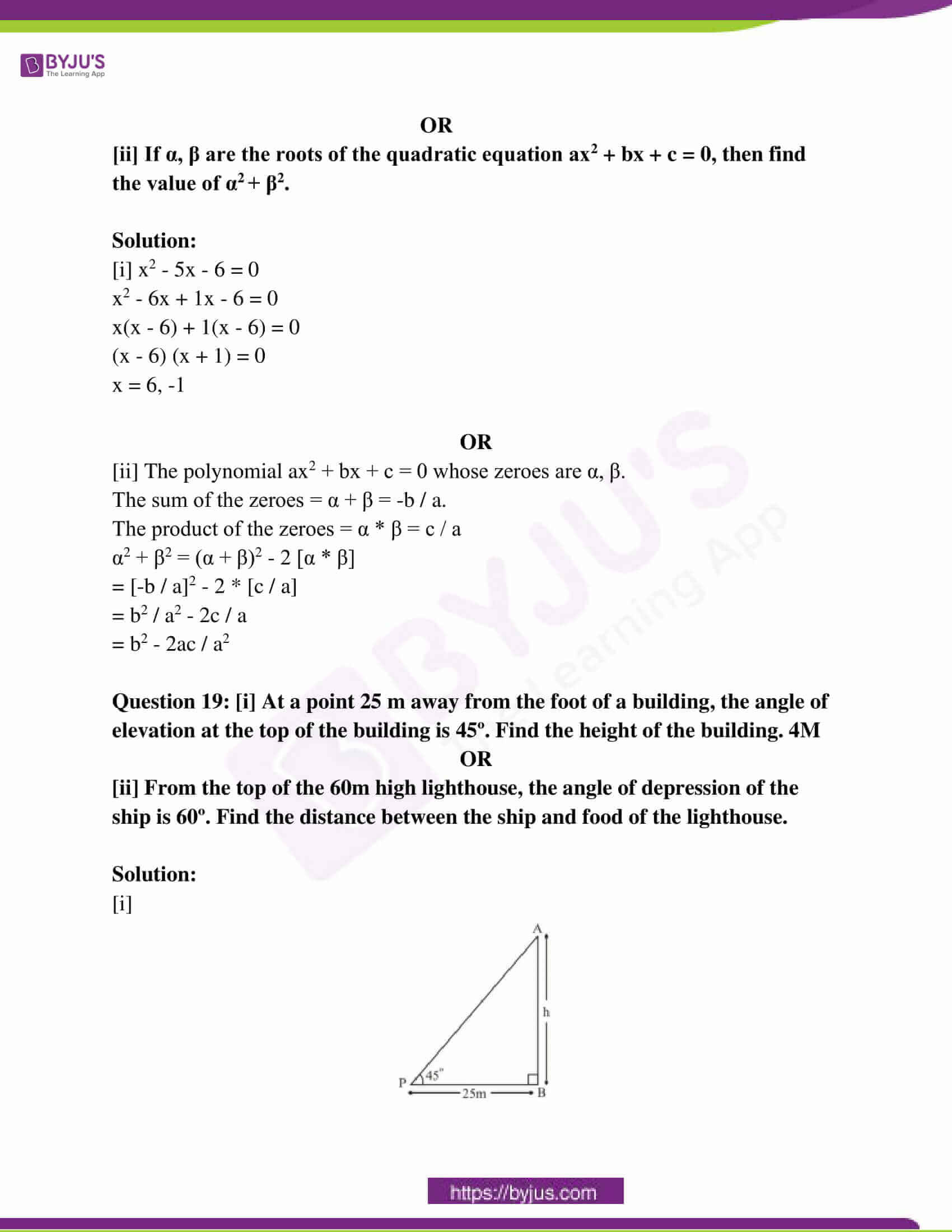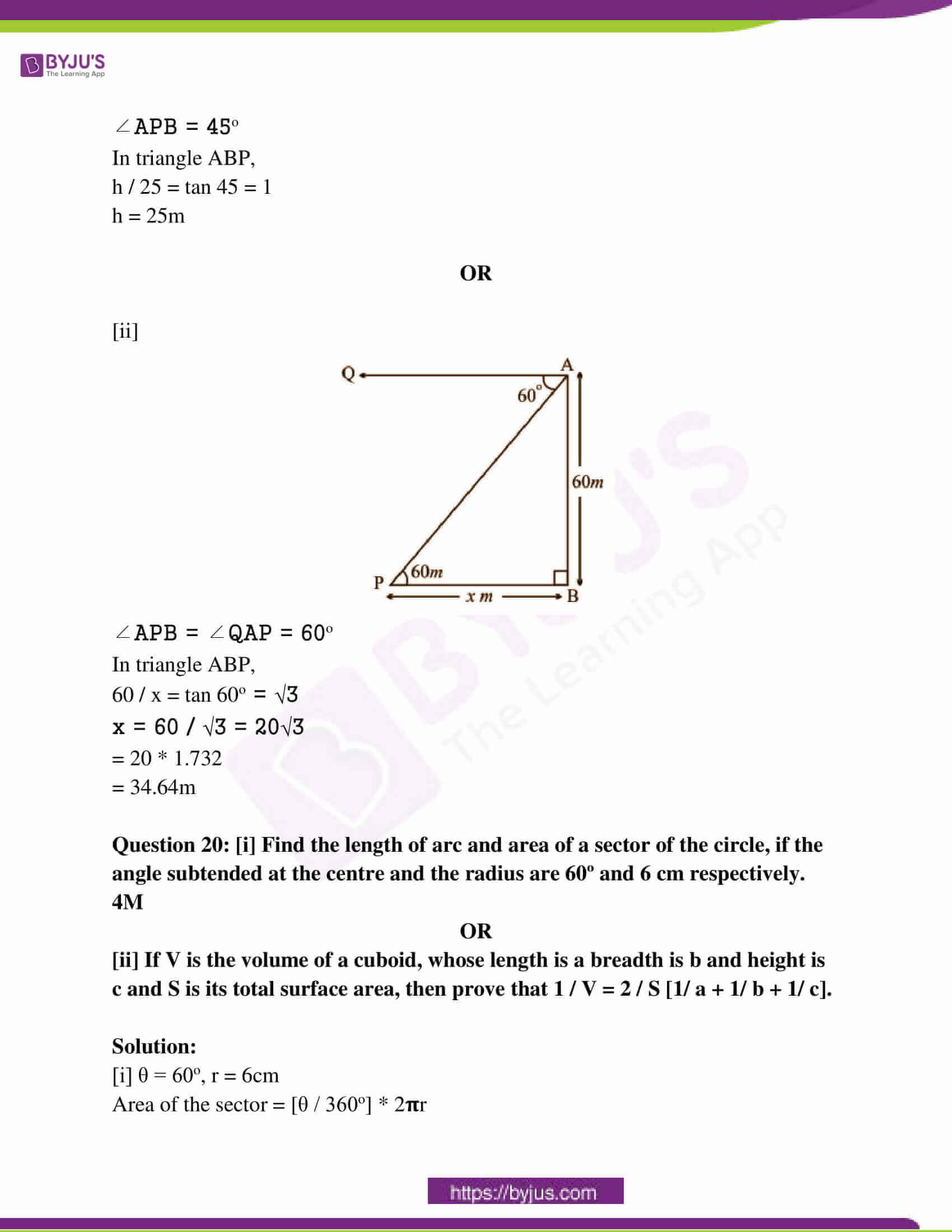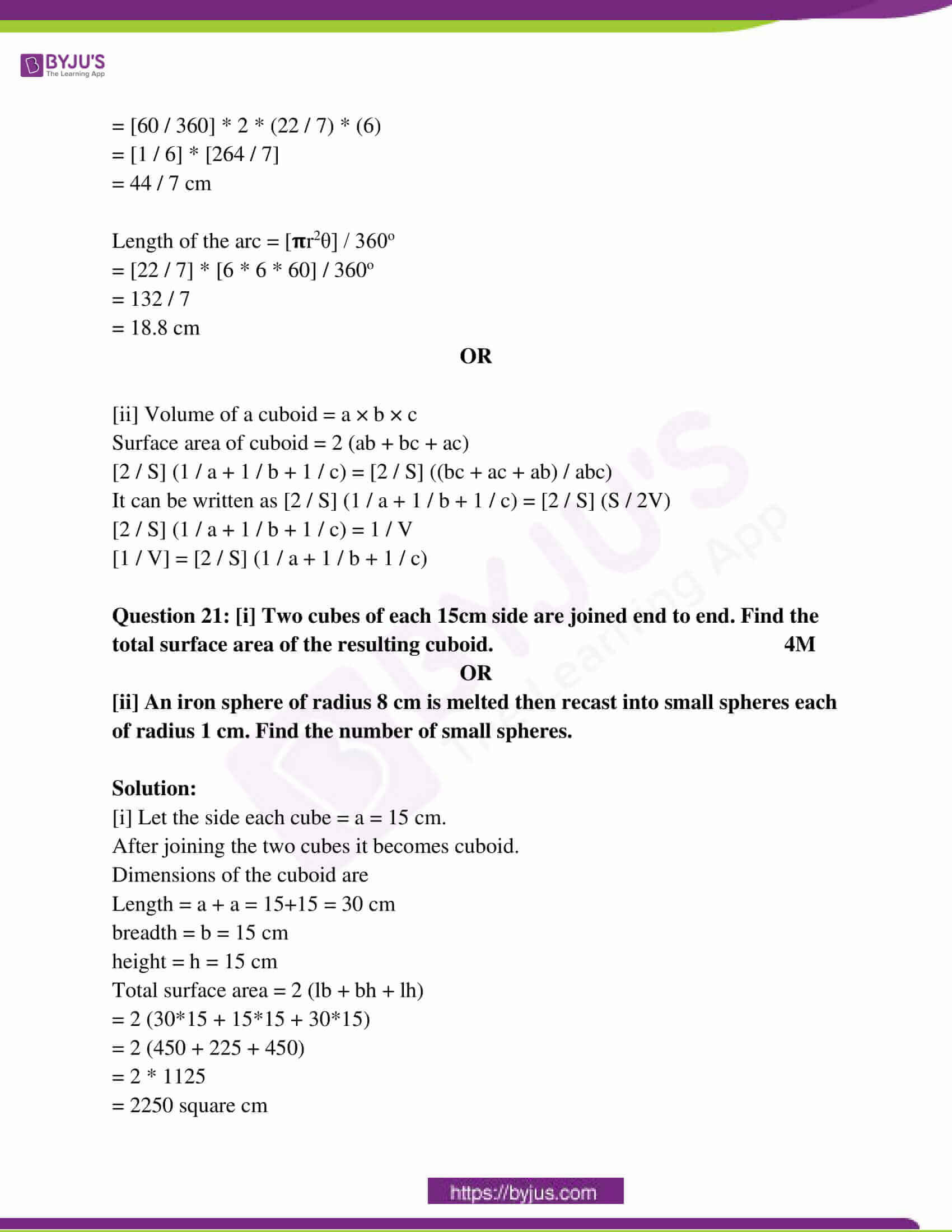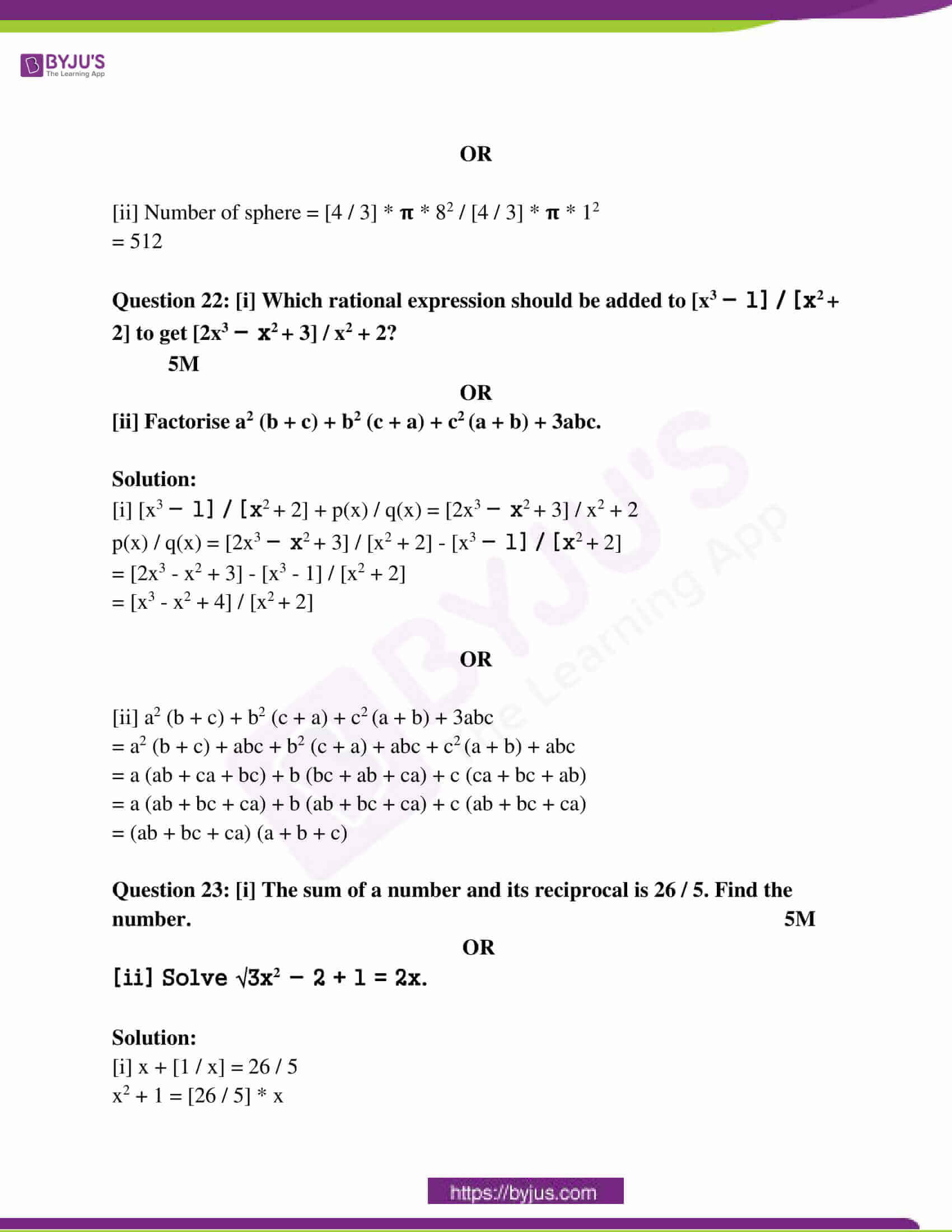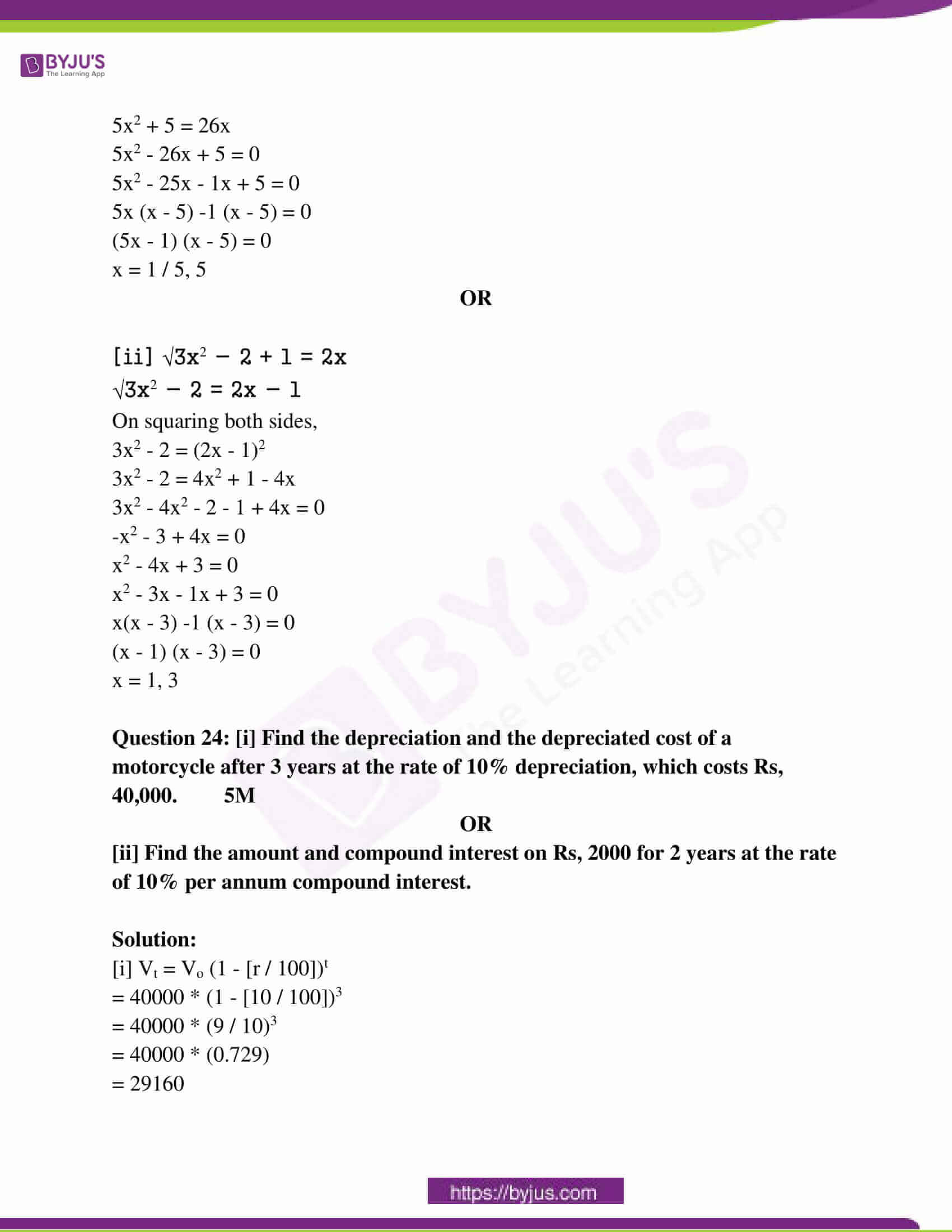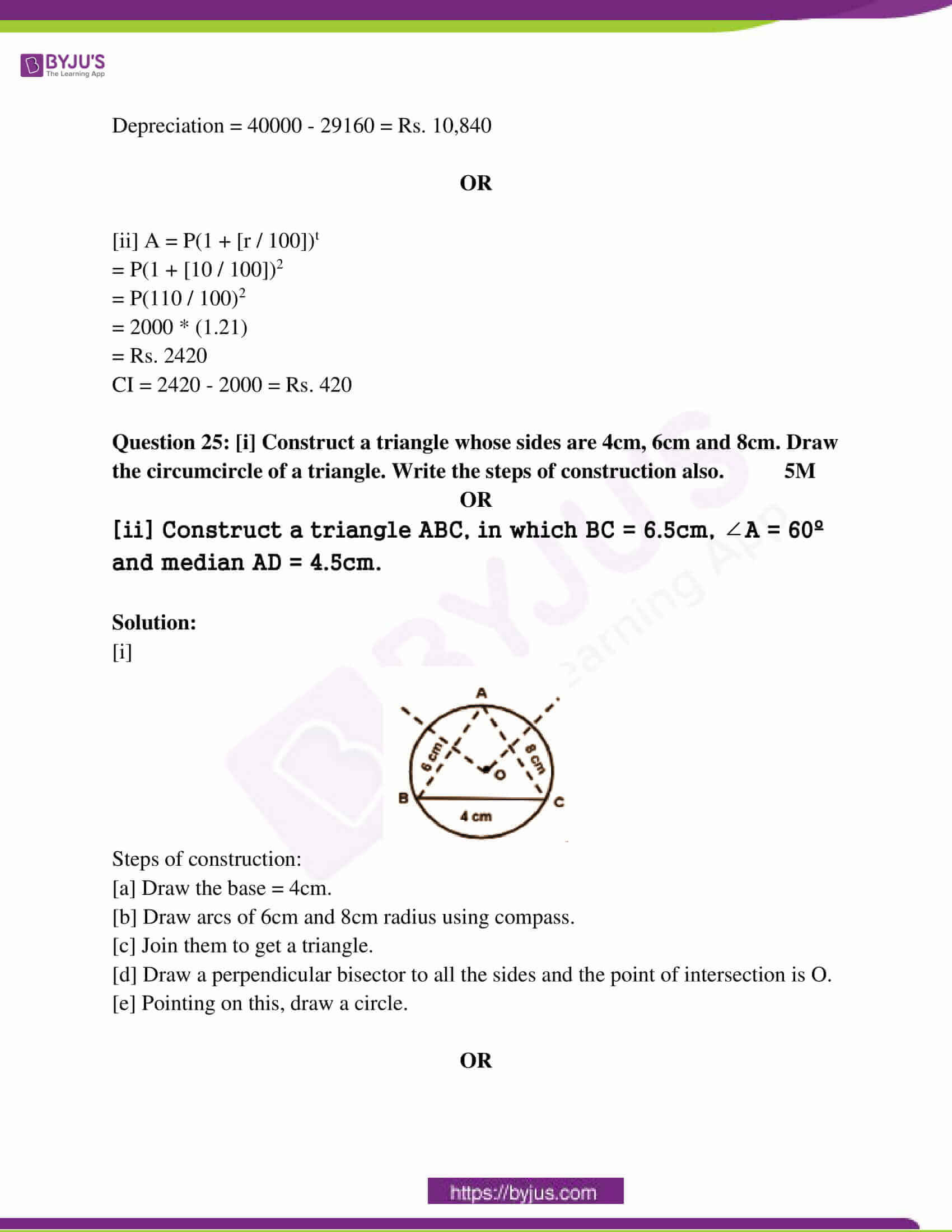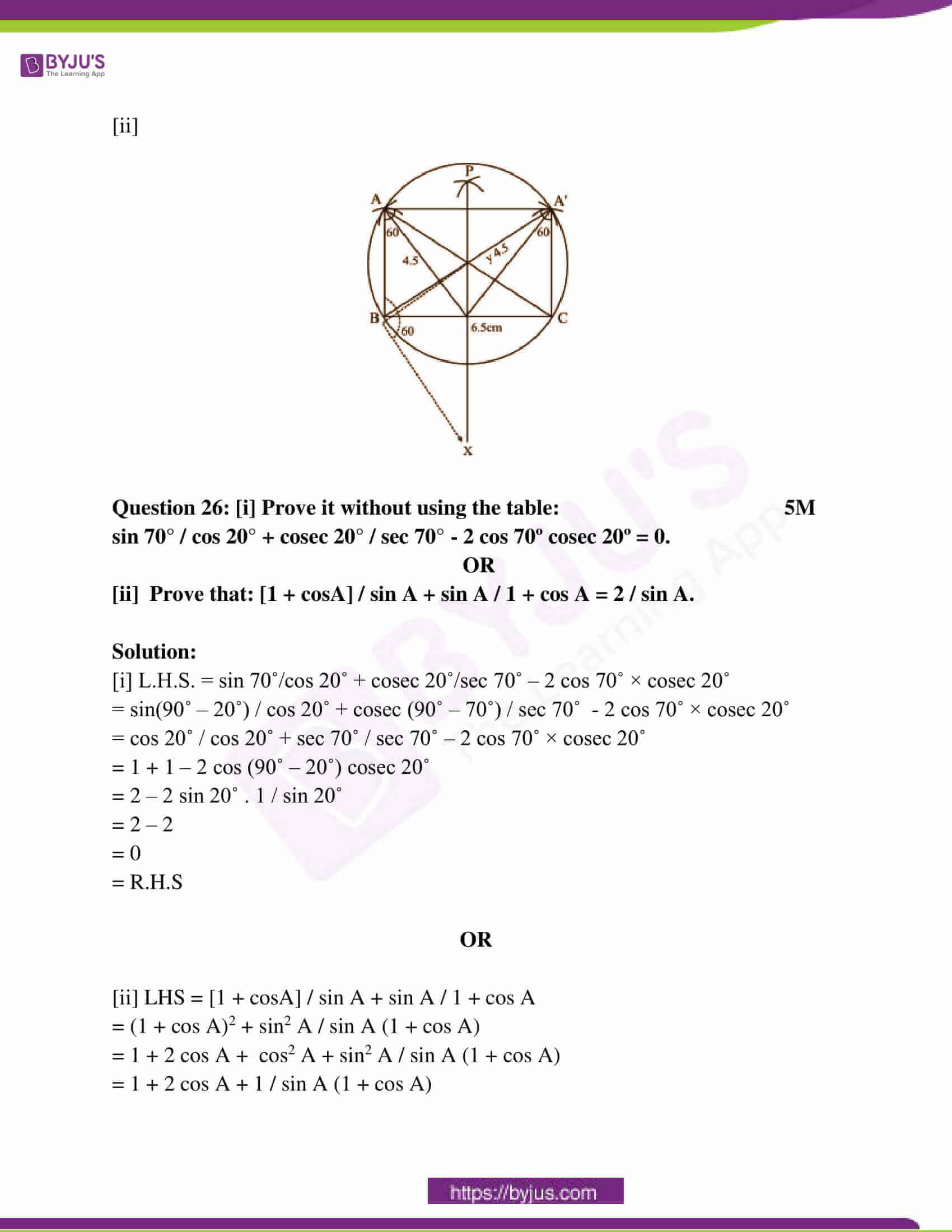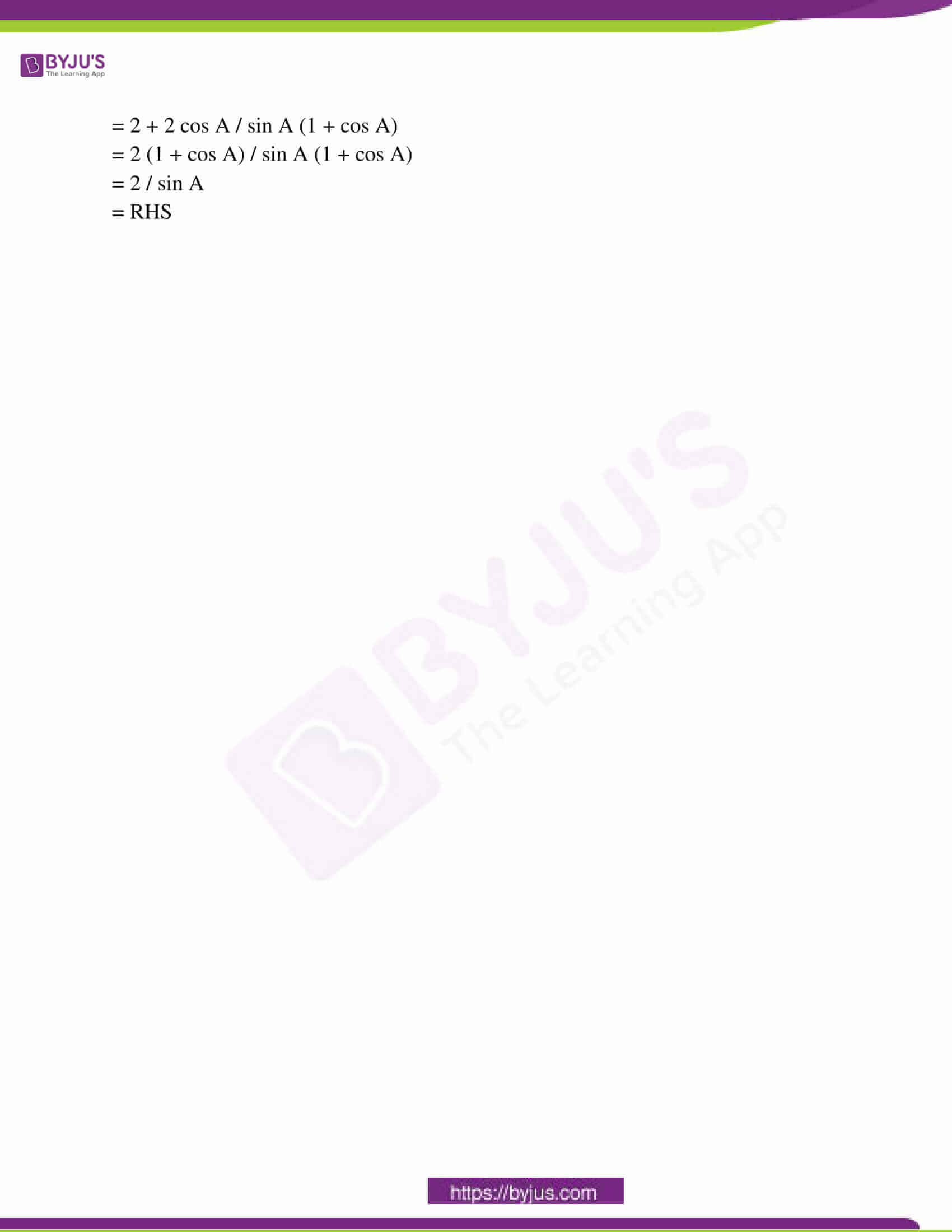(1 * 5 = 5)

Question 1: Choose the correct option and write it in your answer book.

(i) When a1 / a2 = b1 / b2 = c1 / c2, then the system of equations a1x + b1y + c1 = 0 and a2x + b2y + c2 = 0

(a) Has two solutions

(b) Has no solution

(c) Has infinitely many solutions

(d) Has unique solution

(ii) In equation 3x + 2y = 7, then, write the value of x.

(a) 1 (b) 2 (c) -1 (d) 3

(iii) The discriminant of the quadratic equation x2 – 7x – 5 = 0 is

(a) 17 (b) 69 (c) 29 (d) 20

(iv) All squares are

(a) Congruent (b) Equal (c) Similar (d) All of the above

(v) The sum of the pairs of opposite angles of a cyclic quadrilateral is

(a) 360° (b) 90° (c) 180° (d) 270°

Question 2: Write True/ False in the following: (1 * 5 = 5)

(i): Algebraic expression x2 + 3√x – 4 is not a polynomial. [True]

(ii): Property tax is an indirect tax. [True]

(iii): The line joining the centre of a circle to the midpoint of a chord is perpendicular to the chord. [True]

(iv): The volume of cuboid = Length x Breadth x Height. [True]

(v): 2 is the mode of the following observation:

2, 3, 4, 2, 12, 9, 8, 9, 6, 9, 5, 9. [False]

Question 3: Fill in the blanks: (1 * 5 = 5)

(i) Third proportional of 8, 12 is ________________ (18)

(ii) Compound amount = Principle × [1 + _____ / 100] (rate)

(iii) Congruent figures are ____________ (similar)

(iv) If two chords of a circle are equidistant from the center of the circle, then they are ___________ to each other. (equal)

(v) The probability of any sure event is always___________ (1)

Question 4: Write the answer in one word/ sentence of each: (1 * 5 = 5)

(i) Additive inverse of x – [1 / x] is

Answer: – x + [1 / x]

(ii) log m / n =

Answer: log m – log n

(iii) The edge of a cube is 5cm. What will be its volume?

Volume = a3

= 53

= 5 * 5 * 5

= 125 cm3

(iv) The relation between the arc of a circle, the angle subtended by the arc at the centre and radius is

(v) Define Angle of Elevation.

Answer: Angle of elevation is the angle subtended by the line of sight with the horizontal.

Question 5: Match the following. (5 * 1 = 5)

Column A Column B

(i) sin2 45o + cos2 45o (a) 0

(ii) cot (90o – θ) (b) cosec2 θ

(iii) cos θ cos (90 – θ) – sin θ sin (90 – θ) (c) √3 / 2

(iv) 1 + cot2 θ (d) 1

(v) cos 30o (e) cos θ

(f) tan θ

(g) 2

(i) – (d)

(ii) – (f)

(iii) – (a)

(iv) – (b)

(v) – (c)

Question 6: [i] What is the meaning of similarity? 2M

OR

[ii] What are the conditions for the similarity of polygons?

Solution:

[i] Two figures are said to be similar if they both have the same shape, or one has the same shape as the mirror image of the other.

OR

[ii] Two polygons are similar if the corresponding angles are congruent and the corresponding sides are proportional.

Question 7: [i] The perimeters of two similar triangles are 30cm and 20cm respectively. If one side of the triangle is 12 cm, then find the length of the corresponding side of the other triangle. 3M

OR

[ii] Write the statement of Basic Proportionality Theorem (Thales Theorem).

Solution:

[i] Let triangle ABC and triangle PQR are two similar triangles.

⇒ Perimeter of △ABC = 30 cm

⇒ Perimeter of △PQR = 20 cm

⇒ BC = 12 cm, QR = ?

⇒ In the similarity case,

Perimeter of △ABC / Perimeter of △PQR = AB / PQ = BC / QR = AC / PR

[Ratio of their corresponding sides]

⇒ 30 / 20 = 12 / x

⇒ 1.5 = 12 / x

⇒ x * 1.5 = 12

⇒ x = 8cm

OR

[ii] If a line is drawn parallel to one side of a triangle intersecting the other two sides in distinct points, then the other two sides are divided in the same ratio.

Question 8: [i] Check whether 6cm, 8cm and 10cm are the sides of the right-angled triangle? 2M

OR

[ii] ∆ACB is an isosceles triangle such that AC = BC, if AB2 = 2AC2, then prove that ∆ACB is a right-angled triangle.

Solution:

[i] In a right-angled triangle,

10² = 8² + 6²

100 = 64 + 36

100 =100

These are the sides of a right-angled triangle.

OR

[ii]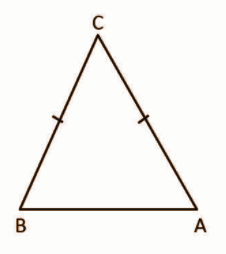AB2 = 2AC2

AB2 = AC2 + AC2

AB2 = AC2 + BC2

So, AB will be the largest side which is the hypotenuse.

AB2 = AC2 + BC2

LHS = AB2

RHS = AC2 + AC2

= 2AC2

= AB2

LHS = RHS

Pythagoras theorem holds good and thus triangle ABC is a right-angled triangle.

Question 9: [i] Find the median of the following values:

15, 35, 18, 26, 19, 25, 29, 20, 27. 2M

OR

[ii] Write any two merits of the Arithmetic Mean.

Solution:

[i] 15, 18, 19, 20, 25, 26, 27, 29, 35

Number of terms = 9

Median = [(n + 1) / 2]th term

= [(9 + 1) / 2]th term

= 5th term

= 25

OR

[ii] (a) It can be easily calculated.

(b) It is based on all observations of the given data.

Question 10: [i] Find the probability that an even number turns up in a single throw of a die. 2M

OR

[ii] Find the probability of getting head and tail at a time in a single throw of a coin.

Solution:

[i] S = {1, 2, 3, 4, 5, 6}

n (S) = 6

A = {2, 4, 6}

n (A) = 3

P (A) = n (A) / n (S)

= 3 / 6

= 1 / 2

OR

[ii] Sample space = {H, T}

Probability (getting head and tail at a time in a single throw of a coin) = 0

Question 11: [i] A chord of length 30cm is drawn at a distance of 8cm from the centre of the circle. Find the radius of a circle. 3M

OR

[ii] Prove that the length of two tangents drawn from an external point to a circle is equal.

Solution:

[i]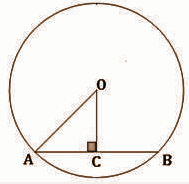Chord of length 30 cm

Given that the distance from centre to the chord AB is OC = 8 cm.

OC ⊥ AB and AC = CB [Since perpendicular drawn from the centre of the circle bisects the chord]

∴ AC = CB = 15 cm

In right ΔOCA,

OA2 = AC2 + OC2

= 152 + 82

= 225 + 64

= 289

AC = 17 cm

Thus, the radius of the circle is 17 cm.

OR

[ii]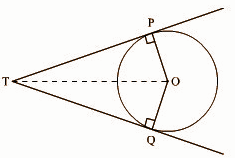Let two tangents PT and QT be drawn to the circle of centre O as shown in the figure.

Both the given tangents PT and QT touch to the circle at P and Q respectively.

To prove that the length of PT = length of QT.

Construction: Draw a line segment, from centre O to external point T {touching point of two tangents}.

Proof: In ∆POT and ∆QOT, the tangent makes a right angle with the radius of the circle.

So, ∠OPT = ∠OQT = 90°

It is clear that both the triangles ∆POT and ∆QOT are right-angled triangles with a common hypotenuse OT of these [as shown in the figure].

In ∆POT and ∆QOT,

∠OPT = OQT = 90°

Common hypotenuse OT and OP = OQ [OP and OQ are radii]

So, by RHS rule of similarity,

∆POT ~ ∆QOT

OP / OQ = PT / QT = OT / OT

PT / QT = 1

PT = QT

Question 12: [i] Prove that the angles in the same segment of a circle are equal to each other. 3M

OR

[ii] Prove that the angles in the semicircle are the right angle.

Solution:

[i]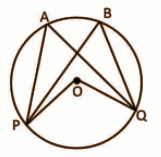Given a circle with centre O, the points P and Q subtends the angles ∠PAQ and ∠PBQ at points A and B respectively.

To prove that ∠PAQ = ∠PBQ

Proof:

Chord PQ subtends ∠POQ at the centre.

From the theorem “Angles subtended by an arc at the centre is double the angles subtended by it at any other point on the circle.”

∠POQ = 2 ∠PAQ —– (1)

∠POQ = 2∠PBQ —– (2)

From (1) and (2),

2∠PBQ = 2∠PAQ

∠PBQ = ∠PAQ

OR

[ii]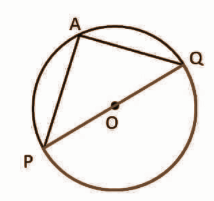Given: A circle with centre O. PQ is the diameter of the circle subtending ∠PAQ at point A on the circle.

To prove that: ∠PAQ = 90o

Proof:

POQ is a straight line passing through centre O.

Angle subtended by arc PQ at O is ∠POQ = 180o —- (1)

Also, by theorem “The angle subtended by an arc at the centre is double the angle subtended by it at any point on the remaining part of the circle.”

∠POQ = 2∠PAQ

∠POQ / 2 = ∠PAQ

180o / 2 = ∠PAQ —- (from (1))

90o = ∠PAQ

∠PAQ = 90o

Question 13: [i] If the mean of 5 observations x, x + 2, x + 4, x + 6, x + 8 is 11, then find the value of x.

OR

[ii] Find the arithmetic mean of 47, 53, 49, 60, 39, 42, 53, 52, 50, 55. 3M

Solution:

[i] x + x + 2 + x + 4 + x + 6 + x + 8 / 5 = 11

5x + 20 = 55

5x = 35

⇒ x = 7

OR

[ii] Mean = ∑x / n

= [47 + 53 + 49 + 60 + 39 + 42 + 53 + 52 + 50 + 55] / 10

= 503 / 10

= 50.3

Question 14: [i] Find the median of the following frequency distribution table:

 Variable (X) 4 6 8 10 12 14 16 Frequency (F) 2 4 5 3 2 1 4

OR

[ii] Write two merits and one demerit of the median. 3M

Solution:

[i]
 Variable (X) 4 6 8 10 12 14 16 Frequency (F) 2 4 5 3 2 1 4 Cumulative frequency(CF) 2 6 11 14 16 17 21

N = 21

Median = [(N + 1) / 2]th term

= [21 + 1 ] / 2

= 22 / 2

= 11th term

= 8

OR

[ii] The two merits of the median are:

(a) It is easy to compute and understand.

(b) It is well defined an ideal average should be.

Demerits :

1) For computing, median data needs to be arranged in ascending or descending order.

Question 15: [i] Solve the following system of equation by Substitution Method: 4x+ y = 7

3x – 2y = 11 4M

OR

[ii] If in ∆ABC, ∠C = 2∠B = ∠A + ∠B + 20, then find all the three angles of the triangle.

Solution:

[i] 4x + y = 7 —- (1)

3x – 2y = 11 —- (2)

From (1), y = 7 – 4x —- (3)

Substitute (3) in (2),

3x – 2 * (7 – 4x) = 11

3x – 14 + 8x = 11

11x = 11 + 14

11x = 25

x = 25 / 11

Put the value of x in (1),

4 * (25 / 11) + y = 7

100 / 11 + y = 7

y = 7 – (100 / 11)

= [77 – 100] / 11

= -23 / 11

So,

x = 25 / 11 and y = -23 / 11

OR

[ii] ∠C = 2∠B = ∠A + ∠B + 20

∠A + ∠B + ∠C = 180o

Since ∠C = 2∠B and 2∠B = ∠A + ∠B + 20,

∠B = ∠A + 20

∠A + ∠B + 2∠B = 180o

∠A + ∠A + 20 + 2 [∠A + 20] = 180o

∠A + ∠A + 20 + 2∠A + 40 = 180

4∠A = 180 – 60

4∠A = 120

∠A = 120 / 4

∠A = 30o

∠B = ∠A + 20

∠B = 30 + 20

∠B = 50o

∠C = 2∠B = 2 * 50o = 100o

Question 16: [i] A fraction becomes 1 / 4 when 2 is subtracted from its numerator and 3 is added to its denominator and on adding 6 to the numerator and multiplying the denominator by 3, it becomes 2 / 3. Find the fraction. 4M

OR

[ii] The sum of two numbers is 7. If the sum of these numbers is 7 times of its difference, then find the numbers.

Solution:

[i] Let the numerator be ‘a’ and denominator be ‘b’.

Given, 3 is added to the denominator and 2 is subtracted from the numerator a fraction becomes 1/4.

⇒ [a – 2] / [b + 3] = [1 / 4]

⇒ 4a – 8 = b + 3

⇒ 4a – b = 11—-(1)

Also, when 6 is added to the numerator and the denominator is multiplied by 3, it becomes 2/3.

⇒ [a + 6] / 3b = [2 / 3]

⇒ 3a + 18 = 2b

⇒ a + 6 = 2b —-(2)

On multiplying equation (2) by 4 and subtracting from equation (1),

⇒ 4a – b – 4a – 24 = 11 – 8b

⇒ 7b = 35

⇒ b = 5

Thus, a = 4.

The fraction is 4 / 5.

OR

[ii] Let the numbers be x and y.

x + y = 7………(1)

x + y = 7 (x – y)

6x – 8y = 0

3x – 4y = 0 * 2 —- (2)

4x + 4y = 28 —- (3)

7x = 28

x = 28 / 7

x = 4

4 + y = 7

y = 7 – 4

y = 3

Question 17: [i] If b is the mean proportional of a and c then prove that [a2 + b2] / ab = [a + c] / b 4M

OR

[ii] If x / b + c = y / c + a = z / a + b, then prove that (b – c) x + (c – a) y + (a – b) z = 0.

Solution:

[i] If b is the mean proportional of a and c, then b2 = ac.

Take the LHS

= [a2 + b2] / ab

= [a2 + ac] / ab

= a (a + c) / ab

= (a + c) / b

OR

[ii] Let x / b + c = y / c + a = z / a + b = k.

x = k (b + c), y = k (c + a), z = k (a + b)

LHS = (b – c) x + (c – a) y + (a – b) z

= (b – c) (k (b + c)) + (c – a) (k (c + a)) + (a – b) (k (a + b))

= k (b2 – c2) + k (c2 – a2) + k (a2 – b2)

= k (b2 – c2 + c2 – a2 + a2 – b2)

= k * 0

= 0

Question 18: [i] Solve the following equation by the formula method, x2 – 5x – 6 = 0. 4M

OR

[ii] If α, β are the roots of the quadratic equation ax2 + bx + c = 0, then find the value of α2 + β2.

Solution:

[i] x2 – 5x – 6 = 0

x2 – 6x + 1x – 6 = 0

x(x – 6) + 1(x – 6) = 0

(x – 6) (x + 1) = 0

x = 6, -1

OR

[ii] The polynomial ax2 + bx + c = 0 whose zeroes are α, β.

The sum of the zeroes = α + β = -b / a.

The product of the zeroes = α * β = c / a

α2 + β2 = (α + β)2 – 2 [α * β]

= [-b / a]2 – 2 * [c / a]

= b2 / a2 – 2c / a

= b2 – 2ac / a2

Question 19: [i] At a point 25 m away from the foot of a building, the angle of elevation at the top of the building is 45º. Find the height of the building. 4M

OR

[ii] From the top of the 60m high lighthouse, the angle of depression of the ship is 60º. Find the distance between the ship and food of the lighthouse.

Solution:

[i]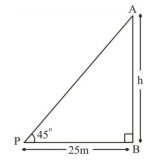∠APB = 45o

In triangle ABP,

h / 25 = tan 45 = 1

h = 25m

OR

[ii]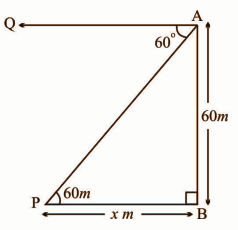∠APB = ∠QAP = 60o

In triangle ABP,

60 / x = tan 60o = √3

x = 60 / √3 = 20√3

= 20 * 1.732

= 34.64m

Question 20: [i] Find the length of arc and area of a sector of the circle, if the angle subtended at the centre and the radius are 60º and 6 cm respectively. 4M

OR

[ii] If V is the volume of a cuboid, whose length is a breadth is b and height is c and S is its total surface area, then prove that 1 / V = 2 / S [1/ a + 1/ b + 1/ c].

Solution:

[i] θ = 60o, r = 6cm

Area of the sector = [θ / 360o] * 2𝛑r

= [60 / 360] * 2 * (22 / 7) * (6)

= [1 / 6] * [264 / 7]

= 44 / 7 cm

Length of the arc = [𝛑r2θ] / 360o

= [22 / 7] * [6 * 6 * 60] / 360o

= 132 / 7

= 18.8 cm

OR

[ii] Volume of a cuboid = a × b × c

Surface area of cuboid = 2 (ab + bc + ac)

[2 / S] (1 / a + 1 / b + 1 / c) = [2 / S] ((bc + ac + ab) / abc)

It can be written as [2 / S] (1 / a + 1 / b + 1 / c) = [2 / S] (S / 2V)

[2 / S] (1 / a + 1 / b + 1 / c) = 1 / V

[1 / V] = [2 / S] (1 / a + 1 / b + 1 / c)

Question 21: [i] Two cubes of each 15cm side are joined end to end. Find the total surface area of the resulting cuboid. 4M

OR

[ii] An iron sphere of radius 8 cm is melted then recast into small spheres each of radius 1 cm. Find the number of small spheres.

Solution:

[i] Let the side each cube = a = 15 cm.

After joining the two cubes it becomes cuboid.

Dimensions of the cuboid are

Length = a + a = 15+15 = 30 cm

breadth = b = 15 cm

height = h = 15 cm

Total surface area = 2 (lb + bh + lh)

= 2 (30*15 + 15*15 + 30*15)

= 2 (450 + 225 + 450)

= 2 * 1125

= 2250 square cm

OR

[ii] Number of sphere = [4 / 3] * 𝛑 * 82 / [4 / 3] * 𝛑 * 12

= 512

Question 22: [i] Which rational expression should be added to [x3 − 1] / [x2 + 2] to get [2x3 − x2 + 3] / x2 + 2? 5M

OR

[ii] Factorise a2 (b + c) + b2 (c + a) + c2 (a + b) + 3abc.

Solution:

[i] [x3 − 1] / [x2 + 2] + p(x) / q(x) = [2x3 − x2 + 3] / x2 + 2

p(x) / q(x) = [2x3 − x2 + 3] / [x2 + 2] – [x3 − 1] / [x2 + 2]

= [2x3 – x2 + 3] – [x3 – 1] / [x2 + 2]

= [x3 – x2 + 4] / [x2 + 2]

OR

[ii] a2 (b + c) + b2 (c + a) + c2 (a + b) + 3abc

= a2 (b + c) + abc + b2 (c + a) + abc + c2 (a + b) + abc

= a (ab + ca + bc) + b (bc + ab + ca) + c (ca + bc + ab)

= a (ab + bc + ca) + b (ab + bc + ca) + c (ab + bc + ca)

= (ab + bc + ca) (a + b + c)

Question 23: [i] The sum of a number and its reciprocal is 26 / 5. Find the number. 5M

OR

[ii] Solve √3x2 − 2 + 1 = 2x.

Solution:

[i] x + [1 / x] = 26 / 5

x2 + 1 = [26 / 5] * x

5x2 + 5 = 26x

5x2 – 26x + 5 = 0

5x2 – 25x – 1x + 5 = 0

5x (x – 5) -1 (x – 5) = 0

(5x – 1) (x – 5) = 0

x = 1 / 5, 5

OR

[ii] √3x2 − 2 + 1 = 2x

√3x2 − 2 = 2x – 1

On squaring both sides,

3x2 – 2 = (2x – 1)2

3x2 – 2 = 4x2 + 1 – 4x

3x2 – 4x2 – 2 – 1 + 4x = 0

-x2 – 3 + 4x = 0

x2 – 4x + 3 = 0

x2 – 3x – 1x + 3 = 0

x(x – 3) -1 (x – 3) = 0

(x – 1) (x – 3) = 0

x = 1, 3

Question 24: [i] Find the depreciation and the depreciated cost of a motorcycle after 3 years at the rate of 10% depreciation, which costs Rs, 40,000. 5M

OR

[ii] Find the amount and compound interest on Rs, 2000 for 2 years at the rate of 10% per annum compound interest.

Solution:

[i] Vt = Vo (1 – [r / 100])t

= 40000 * (1 – [10 / 100])3

= 40000 * (9 / 10)3

= 40000 * (0.729)

= 29160

Depreciation = 40000 – 29160 = Rs. 10,840

OR

[ii] A = P(1 + [r / 100])t

= P(1 + [10 / 100])2

= P(110 / 100)2

= 2000 * (1.21)

= Rs. 2420

CI = 2420 – 2000 = Rs. 420

Question 25: [i] Construct a triangle whose sides are 4cm, 6cm and 8cm. Draw the circumcircle of a triangle. Write the steps of construction also. 5M

OR

[ii] Construct a triangle ABC, in which BC = 6.5cm, ∠A = 60º and median AD = 4.5cm.

Solution:

[i]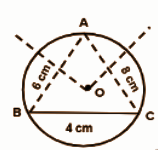Steps of construction:

[a] Draw the base = 4cm.

[b] Draw arcs of 6cm and 8cm radius using compass.

[c] Join them to get a triangle.

[d] Draw a perpendicular bisector to all the sides and the point of intersection is O.

[e] Pointing on this, draw a circle.

OR

[ii]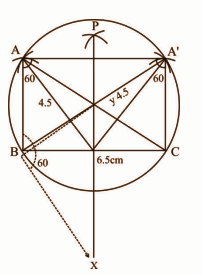Question 26: [i] Prove it without using the table: 5M

sin 70° / cos 20° + cosec 20° / sec 70° – 2 cos 70º cosec 20º = 0.

OR

[ii] Prove that: [1 + cosA] / sin A + sin A / 1 + cos A = 2 / sin A.

Solution:

[i] L.H.S. = sin 70˚/cos 20˚ + cosec 20˚/sec 70˚ – 2 cos 70˚ × cosec 20˚

= sin(90˚ – 20˚) / cos 20˚ + cosec (90˚ – 70˚) / sec 70˚ – 2 cos 70˚ × cosec 20˚

= cos 20˚ / cos 20˚ + sec 70˚ / sec 70˚ – 2 cos 70˚ × cosec 20˚

= 1 + 1 – 2 cos (90˚ – 20˚) cosec 20˚

= 2 – 2 sin 20˚ . 1 / sin 20˚

= 2 – 2

= 0

= R.H.S

OR

[ii] LHS = [1 + cosA] / sin A + sin A / 1 + cos A

= (1 + cos A)2 + sin2 A / sin A (1 + cos A)

= 1 + 2 cos A + cos2 A + sin2 A / sin A (1 + cos A)

= 1 + 2 cos A + 1 / sin A (1 + cos A)

= 2 + 2 cos A / sin A (1 + cos A)

= 2 (1 + cos A) / sin A (1 + cos A)

= 2 / sin A

= RHS